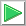# Signal Classification: sine wave or saw tooth.

 Tip When building a training set or a validation set for classification, it is very important to scale all class data to have the same amplitude. For instance, suppose that class 1 is the sine wave and class 2 is the sinc function, one signal must be scaled to make the amplitude of both classes equal. In other words, a significant difference in amplitude in the classes will produce bias, and consequently some preference for one class may be obtained.

 Problem 1 Create a New Project called SignalClass to classify two signals (You must select: Multi-layer Network and Classification in the New Project Dialog). Edit the BuilTrainSet.lab to build an appropriate training set for the classification of two waves: the sine and the saw tooth (see figure). Use 400 training cases and 64 inputs. The input training set must include 200 sine waves and 200 saw tooth waves. Each training case has a wave with a random phase.Solution 1 After editing the file,Run click the button to execute the code. If you do not have any errors, the training set will be generated and displayed on the variable list and the file list. Click on the file to see its contents. The first 100 rows of the training set input are sine waves which random phase, the second 100 rows of the training set input are sawtooth waves. The training set target has two columns, the first column is for class 1 (the sine) and the second column is for class 2 (the saw tooth).

 SignalClass\BuildTrainSet.lab int numCases = 400/2; int numInputs = 64; double deltaInput = 6.28/numInputs; int i; int j; double phase = 0.0; //________________________________ Class 1: sine Matrix inputSine; inputSine.Create(numCases, numInputs); Matrix targetSine; targetSine.Create(numCases, 2); for(i=0; iProblem 2 Edit the BuilValidSet.lab file to build an appropriate validation set for problem 1. Use 200 validation cases and 64 inputs. The validation set input must include 100 sine waves, and 100 saw tooth waves. Each validation case has a wave with random phase.

 Solution 2 After editing the fileRun click the button to execute the code. If you do not have any errors, the validation set will be generated and displayed on the variable list and the file list. Use the scroll bar at the right to visualize each validation case.

 SignalClass\BuildValidSet.lab int numCases = 200/2; int numInputs = 64; double deltaInput = 6.28/numInputs; int i; int j; double phase = 0.0; //________________________________ Class 1: sine Matrix inputSine; inputSine.Create(numCases, numInputs); Matrix targetSine; targetSine.Create(numCases, 2); for(i=0; i

 Problem 3 Edit the Train.lab file to design and train an ANN for the classification of the sine wave and the saw tooth. Use one hidden layer and 2 neurons in this layer. Train the ANN using simulated annealing and the Levenberg Marquardt method.

 Solution 3 After editing the file,Run click the button to execute the code. If you do not have any errors, the ANN will be trained when the code execution stops. Click the network in the variable list to see the network. Then, select the inputTrainSet.csv file, and select the trainSetTarget.csv file. You will be able to see the network behavior in real time by moving the scrollbar at the right.

 SignalClass\Train.lab //_________________________ Network Setup LayerNet net; net.Create(64, 2, 0, 2); // 2 Outputs means 2 Classes int i = 0; //____________________________ Input Scaling for(i = 0; i<64; i++) {      net.SetInScaler(i, -1.0, 1.0); // Input values are from -1 to 1 } //____________________________ Output Scaling net.SetOutScaler(0, 0.0, 1.0); // Output values are from 0 to 1 net.SetOutScaler(1, 0.0, 1.0); // Output values are from 0 to 1 //________________________ Load and set the training set Matrix trainSetInput; trainSetInput.Load(); Matrix trainSetTarget; trainSetTarget.Load(); net.SetTrainSet(trainSetInput, trainSetTarget, false); //________________________ Train net.TrainSimAnneal(100, 100, 15, 0.01, false, 4, 1.0e-12); net.TrainLevenMar(2000,1.0e-12); //_____________________________ Save the trained network net.Save();Problem 4 Edit the CheckTraining.lab file to check the training: (a) Compute the confusion matrix using the training set. (b) Plot the error for each network output. (c) Save the confusion matrix as a vector image (trainConf.emf).

 Solution 4 (a) After editing the file,Run click the button to execute the code. If you do not have any errors, the number of errors will be displayed in the variable list. Click the variable called trainConf to view the confusion matrix for the training set, it should have zero errors as shown below. From this figure, it can be seen that the 200 sine waves and the 200 sawtooth waves were correctly identified.

 SignalClass\CheckTraining.lab //_________________________________________ Load the Training Set Matrix trainSetInput; trainSetInput.Load(); Matrix trainSetTarget; trainSetTarget.Load(); //_________________________________________ Load the ANN LayerNet net; net.Load(); //_________________________________________ Run Matrix output = net.Run(trainSetInput); //_________________________________________ Compute the Confusion Matrix Matrix trainConf = ConfusionMatrix(output, trainSetTarget, 0.5); trainConf.Save(); //_________________________________________ Compute the Number of Errors int numErrors = toint(trainConf.GetSum()) - toint(trainConf.GetDiagonalSum());Problem 5 Edit the Validation.lab file to perform the validation of the ANN. (a) Compute the confusion matrix using the validation set. (b) Plot the error for each network output. (c) Save the confusion matrix as a vector image (validConf.emf).

 Solution 5 (a) After editing the file,Run click the button to execute the code. If you do not have any errors, the number of errors will be displayed in the variable list. Click the variable called validConf to view the confusion matrix for the validation set, it should have zero errors. From this figure, it can be seen that the 100 sine waves and the 100 saw tooth wave were correctly identified

 SignalClass\Validation.lab //_________________________________________ Load the validation set Matrix validSetInput; validSetInput.Load(); Matrix validSetTarget; validSetTarget.Load(); //_________________________________________ Load the ANN LayerNet net; net.Load(); //_________________________________________ Run Matrix output = net.Run(validSetInput); //_________________________________________ Compute the confusion matrix Matrix validConf = ConfusionMatrix(output, validSetTarget, 0.5); validConf.Save(); //_________________________________________ Compute the Number of Errors int numErrors = toint(validConf.GetSum()) - toint(validConf.GetDiagonalSum());Problem 6 Generate a report in Microsoft Word. Write some conclusions in the report focusing on the problems that were faced during the simulation and how these problems were or could be solved.